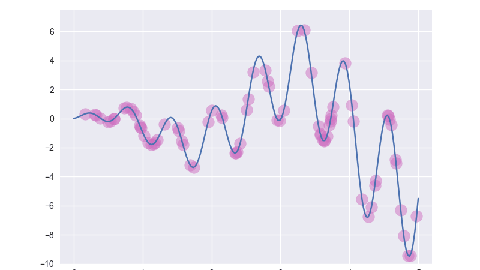# 遗传算法/简单遗传算法## 遗传算法运算过程示意图

``````+------------------------+
|  随机生成初始群体 P(0) |
+-----------+------------+
|
+-----------v------------+
|       个体评价         |
+-----------+------------+
|
+-----------v------------+
|       群体 P(t)        +<-----------------------------------+
+-----------+------------+                                    |
|                                                 |
+-----------v------------+                                    |
|       选择运算         |                                    |
+-----------+------------+                                    |
|                                                 |
+-----------v------------+                         +----------+-----------+
|       交叉运算         |                         |       个体评价       |
+-----------+------------+                         +----------+-----------+
|                                                 ^
+-----------v------------+                                    |
|       变异运算         |                                    |
+-----------+------------+                                    |
|                                                 |
+-----------v------------+                         +----------+-----------+
|      群体 P(t+1)       +------------------------>+        解码          |
+------------------------+                         +----------------------+
``````
• 算法步骤一: 初始化. 随机生成 M 个个体的基因型作为初始群体 P(0). 一种通用的方法是使用固定长度的二进制字符串表示基因.
• 算法步骤二: 计算初始群体的个体适应度. 基因是随机的, 但是"基因是否适应环境"须由算法的设计者进行设计. 评价基因的函数被称为适应度函数, 通常返回一个浮点数, 返回数字越高, 说明该基因越适应环境.
• 算法步骤三: 选择运算. 从当前群体中选择一部分个体进入子代, 个体的适应度越高, 被选取的概率越大.
• 算法步骤四: 交叉运算. 子代个体两两配对, 互相交互部分基因.
• 算法步骤五: 变异运算. 以一定的概率随机改变每个个体的基因.
• 算法步骤六: 终止条件判断. 若未到达终止条件, 回到步骤二; 否则以进化过程中所得到的具有最大适应度的个体作为最优解, 结束算法.

## 遗传算法手工模拟

1. 个体编码 我们使用二进制字符串表示基因, 第一个基因座表示有角和无角, 第二个基因座表示黑色和白色. 因此, 有角白羊可表示为 X = 10, 无角白羊可以表示为 X = 00.
2. 初始群体产生 遗传算法是对群体的进化操作, 需要准备一些表示起始搜索点的初始群体数据. 原则上, 群体越大, 基因越分散越好. 本例中, 群体大小取 4, 初始群体为 [00, 10, 11, 00].
3. 适应度计算 遗传算法以个体适应度的大小评价基因的优劣, 从而决定其遗传机会的大小. 适应度函数需要设计者手工设计, 我们可以假定毛发的颜色比起角的大小来说对山羊的存活更加重要, 此时我们简单的将该群体的适应度表达为 [0.25, 0, 0.5, 0.25].
4. 选择运算 适应度越高的个体传到子代的概率越大, 二号个体此时适应度为 0, 而三号个体此时适应度为 0.5 为最高. 在子代中, 三号个体的基因复制了两遍而二号个体的基因被淘汰. 此时群体为 [00, 11, 11, 00].
5. 交叉运算 个体两两配对, 有一定的概率发生基因的随机交换. 例如, 一号个体和二号个体的基因 00 与 11 发生交叉操作, 变更为 01 与 10. 此时群体为 [01, 10, 11, 00].
6. 变异运算 变异运算对个体的某一个或某一些基因座上的基因值按某一较小的概率进行随机改变, 它是产生新的基因和个体的重要方式. 但是若变异的过于频繁, 也容易失去优秀的个体. 我们假定四号个体的毛色基因发生了变异, 此时群体为 [01, 10, 11, 01].

## 遗传算法的运行参数

• M: 种群大小, 一般取 20-100
• T: 终止进化代数, 一般取 100-500
• PC: 交叉概率, 一般取 0.4-0.99
• PM: 变异概率, 一般取 0.0001-0.1

## 基本遗传算法在函数优化方面的应用举例

: 求 `f(x)=sin(10x) * x + cos(2x) * x` 在 [0, 5] 区间内的最大值.

:

1. 确定基因型的编码与解码方式. 此处使用 10 位二进制编码表示 x 的范围, 即基因型 0000000000 表示 x=0, 基因型 1111111111 表示 x=5.
2. 确定个体评价方法. 此处直接使用 f(x) 作为个体适应度评价.
3. 设定遗传算子运行参数.
``````import matplotlib.animation
import matplotlib.pyplot as plt
import numpy as np

plt.style.use('seaborn')

class GA:
def __init__(self):
self.pop_size = 80
self.max_iter = 20
self.pc = 0.6
self.pm = 0.001
self.dna_size = 10
self.x_bound = [0, 5]

def f(self, x):
return np.sin(10 * x) * x + np.cos(2 * x) * x

def encode(self, x):
a = x / (self.x_bound - self.x_bound) * (2 ** self.dna_size - 1)
a = int(a)
return np.array(list(np.binary_repr(a).zfill(self.dna_size))).astype(np.uint8)

def decode(self, per):
return per.dot(1 << np.arange(self.dna_size)[::-1]) / (2**self.dna_size - 1) * self.x_bound

def perfit(self, per):
x = self.decode(per)
return self.f(x)

def getfit(self, pop):
x = self.decode(pop)
r = self.f(x)
return r

def select(self, pop, fit):
fit = fit - np.min(fit)
fit = fit + np.max(fit) / 2 + 0.001
idx = np.random.choice(np.arange(self.pop_size), size=self.pop_size, replace=True, p=fit / fit.sum())
pop = pop[idx]
return pop

def crosso(self, pop):
for i in range(0, self.pop_size, 2):
if np.random.random() < self.pc:
a = pop[i]
b = pop[i + 1]
p = np.random.randint(1, self.dna_size)
a[p:], b[p:] = b[p:], a[p:]
pop[i] = a
pop[i + 1] = b
return pop

def mutate(self, pop):
mut = np.random.choice(np.array([0, 1]), pop.shape, p=[1 - self.pm, self.pm])
pop = np.where(mut == 1, 1 - pop, pop)
return pop

def evolve(self):
pop = np.random.randint(2, size=(self.pop_size, self.dna_size))
pop_fit = self.getfit(pop)
yield pop, pop_fit
for _ in range(self.max_iter - 1):
chd = self.select(pop, pop_fit)
chd = self.crosso(chd)
chd = self.mutate(chd)
chd_fit = self.getfit(chd)
yield chd, chd_fit
pop = chd
pop_fit = chd_fit

ga = GA()
gaiter = ga.evolve()

fig, ax = plt.subplots()
ax.set_xlim(-0.2, 5.2)
ax.set_ylim(-10, 7.5)
x = np.linspace(*ga.x_bound, 200)
ax.plot(x, ga.f(x))
sca = ax.scatter([], [], s=200, c='#CF6FC1', alpha=0.5)

def update(*args):
pop, _ = next(gaiter)
fx = ga.decode(pop)
fv = ga.f(fx)
sca.set_offsets(np.column_stack((fx, fv)))
# plt.savefig(f'/tmp/img/{args+1:0>2}.png')

ani = matplotlib.animation.FuncAnimation(fig, update, interval=200, repeat=False)
plt.show()
``````